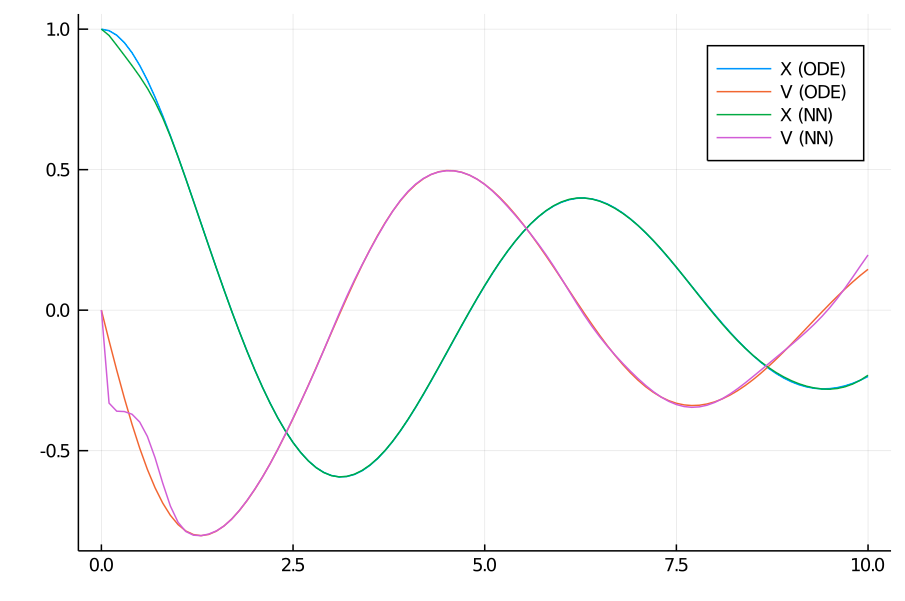# Physics Informed Machine Learning with TensorLayer

In this tutorial, we show how to use the DiffEqFlux TensorLayer to solve problems in Physics Informed Machine Learning.

Let's consider the anharmonic oscillator described by the ODE

$$$ẍ = - kx - αx³ - βẋ -γẋ³.$$$

To obtain the training data, we solve the equation of motion using one of the solvers in DifferentialEquations:

using DiffEqFlux, DifferentialEquations, LinearAlgebra
k, α, β, γ = 1, 0.1, 0.2, 0.3
tspan = (0.0,10.0)

function dxdt_train(du,u,p,t)
du = u
du = -k*u - α*u^3 - β*u - γ*u^3
end

u0 = [1.0,0.0]
ts = collect(0.0:0.1:tspan)
prob_train = ODEProblem{true}(dxdt_train,u0,tspan,p=nothing)
data_train = Array(solve(prob_train,Tsit5(),saveat=ts))

Now, we create a TensorLayer that will be able to perform 10th order expansions in a Legendre Basis:

A = [LegendreBasis(10), LegendreBasis(10)]
nn = TensorLayer(A, 1)

and we also instantiate the model we are trying to learn, "informing" the neural about the ∝x and ∝v dependencies in the equation of motion:

f = x -> min(30one(x),x)

function dxdt_pred(du,u,p,t)
du = u
du = -p*u - p*u + f(nn(u,p[3:end]))
end

α = zeros(102)

prob_pred = ODEProblem{true}(dxdt_pred,u0,tspan,p=nothing)

Note that we introduced a "cap" in the neural network term to avoid instabilities in the solution of the ODE. We also initialized the vector of parameters to zero in order to obtain a faster convergence for this particular example.

Finally, we introduce the corresponding loss function:


x = Array(solve(prob_pred,Tsit5(),p=θ,saveat=ts))
end

loss = sum(norm.(x - data_train))
return loss
end

function cb(θ,l)
@show θ, l
return false
end

and we train the network using two rounds of ADAM:

res1 = DiffEqFlux.sciml_train(loss_adjoint, α, ADAM(0.05), cb = cb, maxiters = 150)
opt = res2.u
using Plots
plot!(ts, data_pred[2,:],label = "V (NN)")